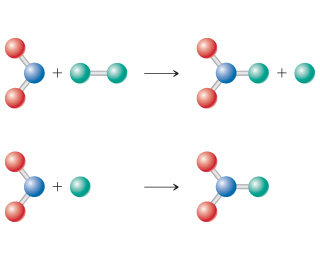Chemistry Practice Problems Reaction Mechanism Practice Problems Solution: Consider the diagram below, which represents two s...

# Solution: Consider the diagram below, which represents two steps in an overall reaction. The red spheres are oxygen, the blue ones nitrogen, and the green ones fluorine.Write the equation for the overall reaction.

###### Problem

Consider the diagram below, which represents two steps in an overall reaction. The red spheres are oxygen, the blue ones nitrogen, and the green ones fluorine.Write the equation for the overall reaction.

###### Solution

We're asked to write the equation for the overall reaction based on the given diagram.

We know that:

• red spheres are oxygen, O
• blue spheres are nitrogen, N
• green ones are fluorine, F

To do so, we need to do these steps:

Step 1.  Write the equation for each given reaction (two steps) based on the diagram

Step 2. Write the equation for the overall reaction by canceling out intermediates

Recall that intermediates are substances that are found on both sides of a chemical equationView Complete Written Solution

Reaction Mechanism

Reaction Mechanism

#### Q. Consider the diagram below, which represents two steps in an overall reaction. The red spheres are oxygen, the blue ones nitrogen, and the green ones ...

Solved • Thu Dec 06 2018 09:35:36 GMT-0500 (EST)

Reaction Mechanism

#### Q. What is the molecularity of each of the following elementary reactions? Write the rate law for the reaction.Cl2 (g) → 2 Cl (g)

Solved • Thu Dec 06 2018 09:35:36 GMT-0500 (EST)

Reaction Mechanism

#### Q. What is the molecularity of each of the following elementary reactions? Write the rate law for the reaction.OCl- (aq) + H2O (l) → HOCl (aq) + OH- (aq)...

Solved • Thu Dec 06 2018 09:35:36 GMT-0500 (EST)

Reaction Mechanism

#### Q. The following diagrams represent mixtures of NO(g) and O2(g). These two substances react as follows:2 NO ( g ) + O2 ( g ) → 2 NO2 ( g )It has been det...

Solved • Thu Dec 06 2018 09:35:31 GMT-0500 (EST)